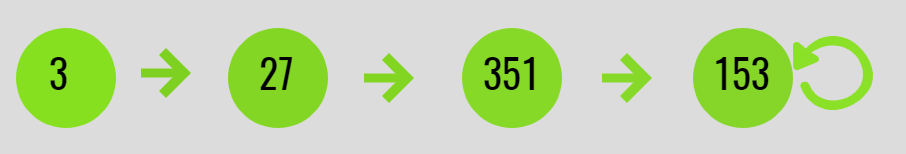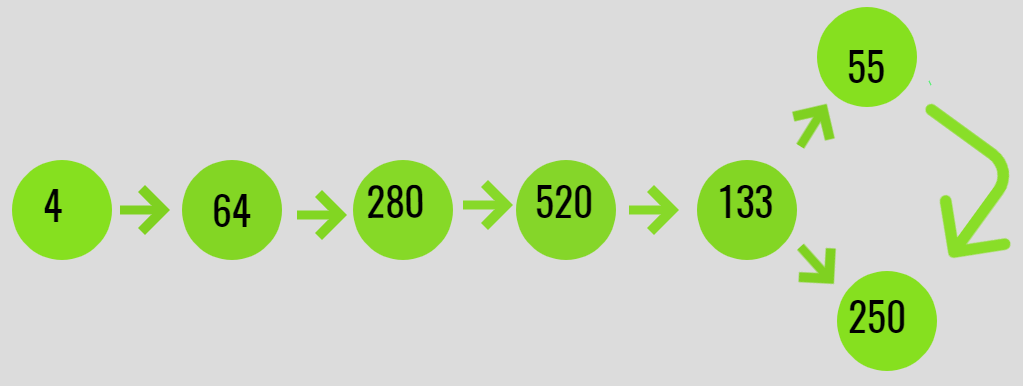Check if digit cube limit of an integer arrives at fixed point or a limit cycle

• Last Updated : 14 Jul, 2021

Given an integer N, the task is to check if the Digit Cube limit of an integer arrives at a fixed point or in a limit cycle.

A Digit Cube Limit is a number which repeatedly arrives at a point if its value is computed as the sum of cubes of its digits, i.e. they have the following properties:

• Arrive at a fixed point if it is an Armstrong number.
• Arrive at a limit cycle if it is repeating in a cycle.

Examples:

Input: N = 3
Output: Reached to fixed point 153
Explanation:
F(3) = 3 * 3 * 3 = 27
F(27) = 2*2*2 + 7*7*7 = 351
F(351) = 3*3*3 + 5*5*5 + 1*1*1 = 153
F(153) = 1*1*1 + 5*5*5 + 3*3*3 = 153
Since a fixed point(= 153) was obtained, which is an Armstrong number of order 3. Below is the illustration:Input: N = 4
Output: Arrived at cycle
Explanation:
F(4) = 4 * 4 * 4 = 64
F(64) = 6 * 6 * 6 + 4 * 4 * 4 = 280
F(280) = 2 * 2 * 2 + 8 * 8 * 8 + 0 * 0 * 0 = 520
F(520) = 5 * 5 * 5 + 2 * 2 * 2 + 0*0*0 = 133
F(133) = 1*1*1 + 3*3*3 + 3*3*3 = 55
F(55) = 5*5*5 + 5*5*5 = 250
F(250) = 5*5*5 + 2*2*2 + 0*0*0 = 133
A cycle between
133 -> 55 -> 250 -> 133 is obtained.
Below is the illustration of the same:Approach: Follow the steps below to solve the problem:

• Create a hashMap to store the sum of the cube of digits of the number while iteration.
• Iterate for the next values for the sum of cube of digits of a number and Check if the next sum of the cube of digits is already present in the hashMap or not.
• If the number is already in the hash-map then, check if the number is an Armstrong number or not. If found to be true, then the number reaches a fixed point.
• Otherwise, If the number is not Armstrong number then continue.

Below is the implementation of the above approach:

C++

 // C++ program for the above approach #include #include  // Define the limit#define limit 1000000000 using namespace std; // Function to get the sum of cube// of digits of a numberlong long F(long long N){    // Convert to string to get sum    // of the cubes of its digits    string str = to_string(N);    long long sum = 0;     for (long long i = 0;         i < str.size(); i++) {        long long val            = int(str[i] - '0');        sum += val * val * val;    }     // return sum    return sum;} // Function to check if the number// arrives at a fixed point or a cyclelong long findDestination(long long N){    // Stores the values obtained    set s;     long long prev = N, next;     // Insert N to set s    s.insert(N);     while (N <= limit) {         // Get the next number using F(N)        next = F(N);         // Check if the next number is        // repeated or not        auto it = s.find(next);        if (it != s.end()) {            return next;        }         prev = next;        s.insert(prev);        N = next;    }    return next;} // Function to check if digit cube// limit of an integer arrives at// fixed point or in a limit cyclevoid digitCubeLimit(long long N){     // N is a non negative integer    if (N < 0)        cout << "N cannot be negative\n";     else {         // Function Call        long long ans            = findDestination(N);         // If the value received is        // greater than limit        if (ans > limit)            cout << "Limit exceeded\n";         // If the value received is        // an Armstrong number        else if (ans == F(ans)) {            cout << N;            cout << " reaches to a"                 << " fixed point: ";            cout << ans;        }         else {            cout << N;            cout << " reaches to a"                 << " limit cycle: ";            cout << ans;        }    }} // Driver Codeint main(){    long long N = 3;     // Function Call    digitCubeLimit(N);     return 0;}

Java

 // Java program for the// above approachimport java.util.*;class GFG{     // Define the limitstatic final int limit = 1000000000;   // Function to get the sum of cube// of digits of a numberstatic int F(int N){  // Convert to String to get sum  // of the cubes of its digits  String str = String.valueOf(N);  int sum = 0;   for (int i = 0;           i < str.length(); i++)  {    int val = (int)(str.charAt(i) - '0');    sum += val * val * val;  }   // return sum  return sum;} // Function to check if the number// arrives at a fixed point or a cyclestatic int findDestination(int N){  // Stores the values obtained  HashSet s = new HashSet<>();   int prev = N, next =0;   // Insert N to set s  s.add(N);   while (N <= limit)  {    // Get the next number    // using F(N)    next = F(N);     // Check if the next number is    // repeated or not    if (s.contains(next))    {      return next;    }     prev = next;    s.add(prev);    N = next;  }  return next;} // Function to check if digit cube// limit of an integer arrives at// fixed point or in a limit cyclestatic void digitCubeLimit(int N){  // N is a non negative integer  if (N < 0)    System.out.print("N cannot be negative\n");  else  {    // Function Call    int ans = findDestination(N);     // If the value received is    // greater than limit    if (ans > limit)      System.out.print("Limit exceeded\n");     // If the value received is    // an Armstrong number    else if (ans == F(ans))    {      System.out.print(N);      System.out.print(" reaches to a" +                       " fixed point: ");      System.out.print(ans);    }     else    {      System.out.print(N);      System.out.print(" reaches to a" +                       " limit cycle: ");      System.out.print(ans);    }  }} // Driver Codepublic static void main(String[] args){  int N = 3;   // Function Call  digitCubeLimit(N);}} // This code is contributed by Rajput-Ji

Python3

 # Python3 program for the above approach # Define the limitLIMIT = 1000000000 # Function to get the sum of cube# of digits of a numberdef F(N: int) -> int:         # Convert to string to get sum    # of the cubes of its digits    string = str(N)    sum = 0         for i in range(len(string)):        val = int(ord(string[i]) - ord('0'))        sum += val * val * val     # Return sum    return sum # Function to check if the number# arrives at a fixed point or a cycledef findDestination(N: int) -> int:         # Stores the values obtained    s = set()     prev = N    next = 0     # Insert N to set s    s.add(N)     while (N <= LIMIT):         # Get the next number using F(N)        next = F(N)         # Check if the next number is        # repeated or not        if next in s:            return next         prev = next        s.add(prev)        N = next     return next # Function to check if digit cube# limit of an integer arrives at# fixed point or in a limit cycledef digitCubeLimit(N: int) -> int:         # N is a non negative integer    if (N < 0):        print("N cannot be negative")     else:         # Function Call        ans = findDestination(N)         # If the value received is        # greater than limit        if (ans > LIMIT):            print("Limit exceeded")         # If the value received is        # an Armstrong number        elif (ans == F(ans)):            print("{} reaches to a fixed point: {}".format(                N, ans))        else:            print("{} reaches to a limit cycle: {}".format(                N, ans)) # Driver Codeif __name__ == "__main__":         N = 3     # Function Call    digitCubeLimit(N) # This code is contributed by sanjeev2552

C#

 // C# program for the// above approachusing System;using System.Collections.Generic;class GFG{     // Define the limitstatic readonly int limit =       1000000000;   // Function to get the sum// of cube of digits of a// numberstatic int F(int N){  // Convert to String to get sum  // of the cubes of its digits  String str = String.Join("", N);  int sum = 0;   for (int i = 0;           i < str.Length; i++)  {    int val = (int)(str[i] - '0');    sum += val * val * val;  }   // return sum  return sum;} // Function to check if the// number arrives at a fixed// point or a cyclestatic int findDestination(int N){  // Stores the values  // obtained  HashSet s =          new HashSet();   int prev = N, next = 0;   // Insert N to set s  s.Add(N);   while (N <= limit)  {    // Get the next number    // using F(N)    next = F(N);     // Check if the next    // number is repeated    // or not    if (s.Contains(next))    {      return next;    }     prev = next;    s.Add(prev);    N = next;  }  return next;} // Function to check if digit cube// limit of an integer arrives at// fixed point or in a limit cyclestatic void digitCubeLimit(int N){  // N is a non negative integer  if (N < 0)    Console.Write("N cannot be negative\n");  else  {    // Function Call    int ans = findDestination(N);     // If the value received is    // greater than limit    if (ans > limit)      Console.Write("Limit exceeded\n");     // If the value received is    // an Armstrong number    else if (ans == F(ans))    {      Console.Write(N);      Console.Write(" reaches to a" +                    " fixed point: ");      Console.Write(ans);    }     else    {      Console.Write(N);      Console.Write(" reaches to a" +                    " limit cycle: ");      Console.Write(ans);    }  }} // Driver Codepublic static void Main(String[] args){  int N = 3;   // Function Call  digitCubeLimit(N);}} // This code is contributed by gauravrajput1

Javascript


Output:
3 reaches to a fixed point: 153

Time Complexity: O(N)
Auxiliary Space: O(N)

My Personal Notes arrow_drop_up